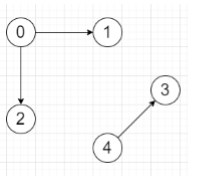# Program to check given graph is a set of trees or not in Python

Suppose we have a graph, represented as a list of edges. We have to check whether the graph is a collection of trees (forest) or not.

So, if the input is likethen the output will be True

To solve this, we will follow these steps −

• Define a function dfs() . This will take node, prev

• if node in seen, then

• return False

• insert node into seen

• for each adjacent node n in e[node], do

• if n is not same as prev, then

• if dfs(n, node) is false, then

• return False

• return True

• From the main method, do the following −

• e := an empty map

• for each start node u and end node v in edges, do

• insert v at the end of e[u]

• insert u at the end of e[v]

• seen := a new set

• for each node in e, do

• if node is not seen and dfs(node, -1) is false, then

• return False

• return True

Let us see the following implementation to get better understanding −

## Example

Live Demo

from collections import defaultdict
class Solution:
def solve(self, edges):
e = defaultdict(list)
for t,f in edges:
e[t].append(f)
e[f].append(t)

seen = set()

def dfs(node, prev):
if node in seen:
return False
return False
return True

for node in e:
if node not in seen and not dfs(node, -1):
return False
return True

ob = Solution()
edges = [[0, 1],[0, 2],[4, 3]]
print(ob.solve(edges))

## Input

[[0, 1],[0, 2],[4, 3]]

## Output

True